2. LINEAR PROGRAMMING

2.1 INTRODUCTION

Linear programming is of one of the most powerful tools of management science. Linear programming (or simply LP) refers to several related mathematical techniques that are used to allocate limited resources among competing demands in an optimal way.

In general, the goal of manager decision making is to achieve some objective such as maximising profit or minimising costs.

A Linear Program (LP) is a problem that can be expressed as follows (the so-called Standard Form):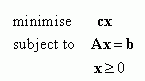(1.1)
where x is the vector of variables to be solved for, A is a matrix of known coefficients, and c and b are vectors of known coefficients. The expression "cx" is called the objective function, and the equations "Ax=b" are called the constraints. All these entities must have consistent dimensions. The matrix A is generally not square, hence you don't solve an LP by just inverting A. Usually A has more columns than rows, and Ax=b is therefore quite likely to be under-determined, leaving great latitude in the choice of x with which to minimise cx.

The set of factors under the control of the manager is called the decision variables. There are also a number of factors that limit or constrain what can be done. These may include, for example, capacities of plant and equipment, limits on market demands, or processing and delivery requirements.

A linear programming model, or LP model, is a particular type of mathematical model in which the relationships involving the variables are linear, and in which there is a single performance measure or objective. An advantage of this type of model is that there exists a mathematical technique, called linear programming that can determine the best or optimal decision even when there are thousands of variables and relationships.

The importance of linear programming derives in part from its many applications (see further below) and in part from the existence of good general-purpose techniques for finding optimal solutions. They are fast and reliable over a substantial range of problem sizes and applications.

2.1.1 Essential conditions

There are five conditions for linear programming to pertain.

• First, there must be limited resources (e.g., a limited number of workers, machines, finances, and material); otherwise there would be no problem, nothing to be sold.
• Second, there must be an explicit objective (such as maximise profit or minimise cost). When a single objective is to be maximised or minimised, we can use linear programming. When multiple objectives exist,goal programming is used.
• Third, there must be linearity (if it takes three hours to make a part, then two parts would take six hours, three parts would take nine hours etc.). Other restrictions on the nature of the problem may require that it be solved by other variations of the technique, such as non-linear programming or dynamic programming.
• Fourth, there must be homogeneity (the products are identical, or all the hours available from a worker are equally productive).
• Fifth is divisibility (products and resources can be subdivided into fractions). If this subdivision is not possible (such as flying half an aeroplane or hiring one-fourth of a person), a modification of linear programming, called integer programming, can be used.
Linear programming does not allow for uncertainty in any of the relationship; there cannot be any probabilities or any random variables. Also any time-dependent changes cannot be involved into classical LP problem.

Since its discovery in the late 1950s, linear programming has been applied to a wide variety of decision problems in business and the public sector.

2.1.2 Basic groups of applications

Product mix 1: Finding the minimum-cost or maximum profit production schedule, including production rate change costs, given constraints on size of workforce and inventory levels. This type of example contains several types of products (agriculture crops, services). Each of them is characterised by different cost and profit and different demand for labour, equipment (machine hours), material, land etc. The task is to find the best combination of product according the target (target function). Generally each combination of product is possible (not all product are usually in optimal solution). If not, special constrains must be added.

Example:Product Planning

Example: Product Planning - LP Model

In fact there are more types of problem, which can be included into the basic type Product  mix but have some special features.

Product mix 2:Finding the optimal combination of product. Each product must be processed sequentially through several machine centres, with each machine in a centre having its own cost and output characteristics.

Example: Product processing

Example: Product processing  LP model

Product mix 3: There are limited sources in different time periods and different demands. The production cost, possible sales and profit also varies by time unit (i.e. week, month).

Example deals with the formulation of a large-scale or system model, designed primarily for planning the integrated activities of a business firm.

Example: Production scheduling

Example: Product processing  LP model

Warehouse problem: The manager of a warehouse buys and sells the stock of a certain commodity, seeking to maximise profit over a period of time, called the horizon. The warehouse has a fixed capacity, and there is a holding cost that increases with increasing levels of inventory held in the warehouse (this could vary period to period). The sales price and purchase costs of the commodity fluctuate. The warehouse is initially empty and is required to be empty at the end of the horizon. This is a variation of the production scheduling problem, except demand is not fixed.

Product blending: Blending problem deals with finding the optimal product mix where several products have different costs and resource requirements (e.g., finding the optimal blend of constituents for gasolines, paints, human diets, animal feeds)

Example : Blending Problem

Example : Blending Problem  LP model

Example : Blending Problem - total formulation.

Service productivity analysis: Comparing how efficiently different service outlets are using their resources compared to the best performing unit. (This approach is called data envelopment analysis).

Process control: Minimising the amount of scrap material generated by cutting steel, leather, or fabric from a roll or sheet or bar of stock material.

Example: Process control

Example: Process control  LP model

Inventory control: Finding the optimal combination of products to stock in a warehouse or store.

Following applications represents independent group of LP models and are described in the chapter transportation methods:

Distribution scheduling: Finding the optimal shipping schedule for distributing products between factories and warehouses or warehouses and retailers.

Plant location studies: Finding the optimal location of a new plant by evaluating shipping costs between alternative locations and supply and demand sources.

Materials handling: Finding the minimum-cost routings of material handling devices between departments in a plant and of hauling materials from a supply yard to work
sites by trucks, with each truck having different capacity and performance capabilities.

2.1.3 Formulation of Linear Programming Problems

Formulating an LP model means translating a business decision problem into LP terminology by defining variables, specifying an objective function, and writing all constraints as equalities or inequalities. This work needs creativity and experience. Basic approach and steps see examples above. The results of model formulation are not necessarily identical for all authors.

Formulating any quantitative model means selecting out the important elements from the problem and defining how these are related. There are some steps that have been found useful in formulating linear programming models. These are:

• Define in verbal terms the objective that you are trying to achieve in solving the problem. Select only one objective (maximal profit or minimal cost or maximal sales etc.).
• List verbally the decisions that are to be made.
• List verbally the constraining factors that affect these decisions. There are several general types of constraints listed below. See if your problem has any of these conditions. Any given problem will generally not have all of the types of constraints.
• Capacity constraints. These are limits because of the amount of equipment, space, or staff available.
• Market constraints. These are limits (either lower or upper limits or both) on how many products can be sold or used.
• Availability constraints. These are limits because of scarcity of raw materials, labour, funds, or other resources.
• Quality or blending constraints. These are constraints that put limits on mixes of ingredients, usually defining the quality of output products.
• Production technology or material balance constraints. These are constraints that define the output of some process as a function of the inputs, often with a loss for scrap.
• Definitional constraints. These are constraints that define a given variable. Often, such constraints come from accounting definitions.
• Define specifically the decision variables. What is needed is a list of variables their definitions, including specification of units of measurement.
• Define the objective function in detail. For each decision variable a cost or profit coefficient must be defined.
2.1.4 Summary

Although linear programming has proven to be a valuable tool in solving large and complex problems in business and the public sector, there are limitations mentioned above. Linear programming is not useful for problems that require integer solutions, that involve uncertainty, development in time, or that have a non-linear objective function or constraints. These limitations indicate that linear programming cannot be applied to all problems. However, for those problems for which it is applicable, it has proven to be a useful and powerful tool.

2.2 SOLUTION OF LINEAR PROGRAMMING PROBLEMS
Since actual linear problems may be large and complex, sophisticated computer methods are used to solve them. But for a manager facing a decision problem, the model solution is only a part of the answer. Analysis using models aims to get a better understanding of the problem, and the effect of various constraints and "what if" questionsin short, sensitivity analysis. The insight gained is often more valuable than the specific numerical answer. One of the advantages of linear programming is that it is very rich in providing such sensitivity information as a direct part of the solution. In order to understand this sensitivity analysis, it is necessary to have some understanding of the solution process.

2.2.1 Graphical solution
It is useful to get familiar with the graphical method for solving LP problems first  not because this is used in practice, but because it provides best understanding of the model and its solution.

Graphical solution is based on a geometrical representation of the feasible region and the objective function. In particular, the space to be considered is the n-dimensional space with each dimension defined by one of the LP variables. The objective function will be described in this n-dim space by its contour plots, i.e., the sets of points that correspond to the same objective value. To facilitate the visualisation we are restricted to the two-dimensional case, i.e., to LP models with two decision variables (possibly with 3 variables in 3D graphic).

The steps of the graphical method are as follows:

1. Formulate the Problem in Mathematical Terms Example: Product planning - LP model

As mentioned above there are

• limited resources
• an explicit objective function
• the equations are linear
• the resources are homogeneous
• the decision variables are divisible and non-negative
2. Plot Constraint Equations

Every vector of two the variables can be plotted using two points with

co-ordinates in a 2-dimensional (planar) Cartesian system. The constraint equations are easily plotted by letting one variable equal zero and solving for the axis intercept of the other. (The inequality portions of the restrictions are disregarded for this step.)

3. Determine the Area of Feasibility

The direction of inequality signs in each con-constraint determines the area of feasibility where a feasible solution is found.
The feasible region of a 2-variables LP is depicted by the set of points the co-ordinates of which satisfy all LP constraints and the sign restrictions. If all constraints are expressed by linear inequalities, to geometrically characterise the feasible region, we must first characterise the set of points that constitute the solution space of each linear inequality. Then, the LP feasible region will result from the intersection of the solution spaces corresponding to each technological constraint.

A feasible solution or feasible point satisfies all of the constraints and any restrictions on the variables value (e.g. nonnegativities). The feasible set is a set of all feasible solutions.

The region of feasible solutions forms a convex polygon. If this condition of convexity does not exist, the problem is either incorrectly set up or not amenable to linear programming.

4. Plot the Objective Function

The objective function may be plotted by assuming some arbitrary total profit figure and then solving for the axis co-ordinates, as was done for the constraint equations. Other terms for the objective function, when used in this context, are the iso-profit line or equal contribution line, because it shows all possible production combinations for any given profit figure. All iso-profit lines are parallel, so that we can move it to one side to maximise the objective function or other side for minimisation.

5.Find the Optimum Point
It can be shown mathematically that the optimal combination of decision variables is always found at an extreme point (corner point) of the convex polygon. The number of corner points is limited (compare with unlimited number of points of the whole polygon representing the set of feasible solutions). We can determine which one is the optimum by either of two approaches.

The first approach is to find the values of the various comer solutions algebraically. This entails simultaneously solving the equations of various pairs of intersecting lines and substituting the quantities of the resultant variables in the objective function.

The second and generally preferred approach entails using the objective function or iso-profit line directly to find the optimum point. The procedure involves simply drawing a straight line parallel to any arbitrarily selected initial iso-profit line so that the iso-profit line is farthest from the origin of the graph (in cost-minimisation problems, the objective would be to draw the line through the point closest to the origin.)

Note that all iso-profit lines have the same slope but different axis intercepts.

The profit lines of a linear programming model are always parallel.

The underlying idea is to keep sliding'' the iso-profit line in the direction of increasing value of the objective function, until we cross the boundary of the LP feasible region. This extreme point of the feasible region is the optimum point.

If there is a finite optimal solution for a linear programming model, an optimal solution will be a corner point.

Not all examples lead to one optimal solution. It could happen that attempting to plot the feasible region for some problem, we get no points in feasible region i.e. on the plane that satisfy all constraints, and therefore the problem is infeasible called also over-constrained problem.

If there is no point in the feasible set, there is no feasible solution of the linear programming model.

One possible reason for no feasible solution is an error in transcribing the data or inputting model. Another type of error that is harder to determine is the inclusion of reasonable constrains, but with no possibility to satisfy them all. For example, there may not be enough available capacity to satisfy all of the requirements.

In the LP modes considered above, the feasible region (if not empty) was a bounded area. For this kind of problems it is obvious that all values of the LP objective function (and therefore the optimal) are bounded.

A bounded feasible set is one which a finite numbers may be specified so that any variable value, at any point in the feasible set, is less or equal to that number.

Consider however the possibility that the feasible region is not bounded and the iso-profit line can be shifted with no limits. An unbounded feasible set is a feasible set that is not bounded. Only one variable has to be limitless for a feasible set to be unbounded. If the feasible point exists with the objective function value as favourable as desired, a model is said to have an unbounded optimal solution and the problem is called unbounded problem (usually one extreme - maximum or minimum can be found, problem is if this is not the extreme we search for). The practical interpretation of such optimal solution is the same as in the previous case i.e. there is no optimal solution and the model creator must return to the real situation and check all constrains. In the unbounded case there is probably some limitation missing, forgotten in the model formulation.

The model can have also more than one optimal solution  alternative solution. In this case the iso-profit line goes in the same direction as one of constrain line. The extreme points than is a set of points lying between two polygon corner points on this line (contour line).

If two corner points are optimal, then all of the points on the line segment connecting them are also optimal.

Note that for all examples of optimal solutions for linear programming model, there is an optimal solution in the corner point.

2.2.2 Simplex method
The method generally used for solving linear programming problems is called the simplex method. George Dantzig originally developed it in 1957.

The method starts with a basic solution, then modifies this basic solution by adding and dropping a variable, always increasing profit (or reducing cost) until an optimal solutions found. It is a search process, but it turns out to be amazingly efficient in solving very big problems.

Comparing with the graphical solution it starts in one corner point (initial basic solution) that moves to another corner point with better value of target function etc. and stops in the corner with the best value of the target function (optimal solution).

The basic of the method is that the optimal solution can be found only in corner points of the feasible area (in the case of alternative optimal solutions on the

The simplex algorithm always starts from the initial simlex tableau containing the initial basic solution (basic solutions corresponds with the corner points in the graphical solutions).

The steps of the simplex method are as follows:
1. Converting all inequalities to equation by introducing slack and surplus variables.

All slack and surplus variables have their interpretations (sometimes including units) as structural variables .The surplus variables are subtracted from the LHS, whereas slack variables are added. The objective function is the same as that of original model. That is, the objective function coefficient for surplus and slack variables is always zero.

Conversion of a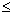Constrain to an = Constrain

A new variable s is introduces to allow the LHS of the converted constrain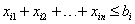(1.2)
to be equivalent to RHS: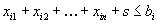(1.3)
The variable s is called slack variable. The constrain (1.3) is equivalent to (1.2) if the slack variable is nonnegative.

Conversion of a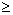constrain to an = constrain
The amount of the LHS is larger than RHS is called the value of surplus variable.
Consider the constrain:(1.4)
Is manipulated to: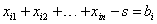(1.5)
A different slack or surplus variable must be introduced for eachandinequality.

2. Conversion of a Constrain with a Negative RHS

It is done by multiplying each constrain coefficient, including RHS constant by 1. If the constrain is an inequality, multiplying by 1 reverses the inequality sign (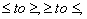). It was verified that this manipulation gives an equivalent model.

3. Initial Solution

If we select some set of the m variables, the rest variables are equal to zero. Then we solve for these m variables and we get have a basic solution. The selected variables are called the solution variables. The variables set equal to zero are the outside variables. In the previous chapter, we found all the basic solutions for this problem are related to corner points of the graphic solution. (Recall that some of the basic solutions are infeasible, since they involve negative values for one or more of the variables.)

The model with m constrains and n variables has m solution variables and (n-m) outside variables. The solution variables have columns under them containing one element with +1, and the remainder of elements 0. The 1 must be in a different row than the 1 of any other column. These columns form a diagonal of 1. If there is less than m such columns we have to add artificial variable (or variables) to get 1 element (in column with all other element equal to 0) on each line (constrain) Example with slack, surplus and artificial variables.

The artificial variable should be added to all constrains with exclusion of those where positive slack variable has been added. The initial solution is written in the initial simplex tableau. All following calculations are provided in following simplex tables.

4. Finding a New Solution

The simplex method finds a new solution by replacing exactly one of the solution variables by one of the outside variables. This is accomplished in three steps:

1. Choose an entering variable that will increase the profitability of the solution.
2. Choose the leaving variable that will ensure a feasible basic solution.
3. Re-solve the constraint equations to find the new solution.

The Entering Variable

Determine the variable that has the greatest net profit per unit. This is the one with the largest positive (negative for minimisation)value in the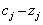row at the bottom of the table. The column r is marked with an arrow.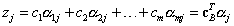(1.6)
Theare the elements of the matrix inside the body of the simplex tableau ( in i-th row and j-th column).

The next step is to determine which of the current solution variables is to leave the solution and be replaced by outside variable xr chosen in previous step. Consider adding units of xr, into the solution one at a time. At some point one of the variables initially in the solution will be driven exactly to zero, hence, changing from a solution variable to an outside variable. We need to find out which solution variable will first be driven to zero as units of xr are introduced into the solution. Divide each amount in the "Solution values" (RHS) column by the amount in the comparable row of the entering xr column.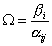(1. 7)
The smallest number obtained by this computation gives the maximum number of units of xr, which may be injected into the solution without driving some solution variable negative. In the same time it identifies the solution variable xs which will leave the basic solution and become outside variable. If any of the amounts calculated by dividing are negative, they should be eliminated from consideration; otherwise, the smallest amount, as computed above, determines the leaving variable.

Note what we have done so far in the simplex procedure. First, we identified a variable that will increase profitability and indicated that it is to be included in the next solution. Then, we guaranteed that the next solution would be a basic feasible one by identifying a variable to be removed from the solution that will keep all variable values nonnegative. The third step is to determine the new solution.

Re-solving the Actual Replacement

The element in the entering xr column and the leaving xs row is designated the pivot element. In order to obtain a new solution containing xr, this element must be converted to a +1 and the other elements in the xr column converted to 0.

To convert the pivot element to + 1 requires that we divide every element in the s-th row by the value of the pivot element. Recall that each row is in reality a constraint equation, and one may divide a whole equation by a constant.

The second part of the procedure is aimed at converting all the elements (except the pivot element) in the r-th column to zero. This can be accomplished by multiplying the entire new s-th row by the value in the xr column, and subtracting the result from the old row (other than s-th).

The result is a new basic solution in a second simplex table. The steps 4 should be repeated till the optimal solution is found.

5. Optimal Solution
If all the coefficient of therow are either zero or negative (positive for minimisation), indicating that no variable can further increase profit if entered in the solution . Hence, an optimal solution has been obtained and the simplex algorithm stops.The graphical overview or summary of the steps of simplex method will help to understand the procedure.

Reading the optimal tableau we should learn

• what are the optimal solution variables and what are their optimal values
• what is the optimal value of the objective function
• is the solution degenerated
• does the alternative optimum exist
and we should be able to formulate the results in text corresponding with the text in the beginning of the whole linear programming procedure.

Another example deals with solving minimisation model.

lt should be noted that in previous problem, we were maximising an amount (profit) subject to constraints, all of which were in the form of a sum of variables equal) to or less than a constant. This is important, since certain steps in the solution process will be modified as we change the description of the problem.

This example will differ from the previous example in several respects:

1. An amount is being minimised (rather than maximised).
2. There are three constraints - one equality and two inequalities.

Note that one of the inequalities is in the form of the variable being equal to or greater than a constant.
To strengthen our understanding of the problem, we shall briefly illustrate graphic analysis and than solve the same problem with the simplex procedure

 algebraic formulation. As in the previous example we get from the minimisation - initial tableau through re-calculations to minimisation  solution.

2.2.3 Special cases
Some special situations encountered in solving linear programming problems are:

• Unbounded solutions, where there is no limit on some entering variable, and hence there is unbounded profitability.
• No feasible solution, when constraints are so restrictive that there is no way of satisfying them.
Both of these can be detected during the simplex procedure. Both generally result from improper formulation of the problem or incorrect data.

Multiple solutions to linear programming problems are possible. This is indicated when one of the outside variables has avalue of zero in the optimal solution.

Degeneracy occurs when one or more of the solution variables equals zero.

Special Situations

Unbounded Solution

In certain situations, it is possible that the linear programming problem does not have a finite solution. For example, assume that the third simplex table appeared as shown(re-calculations, Table 2). Variable x1 is the entering variable. Column x1, has been changed so that not onlyis negative (as it was in Table 2) but all coefficients in the x1, column are negative. ln this situation, there is no finite optimum solution. If profit was being maximised, an analogous situation would occur if thetotal were positive and all coefficients in the column were negative.

If the above situation develops, the cause will frequently be a misstated constraint or incorrect data. Business situations properly described will not usually result in an unbounded solution as illustrated above.

Multiple Solutions

There may be more than one optimal solution to a linear programming problem. In this case, two (or more) basic solutions; will have the same optimum profit (or cost).

In the optimal tableau of example minimisation  solution no; value is negative, indicating an optimum has been reached. Imagine, however, for example variable x4, is not a solution variable and= 0. This indicates that variable x4, can be brought into the solution without increasing or decreasing the cost. The optimal solutions lies in the corner points of feasible area and any convex combination of these corner points is an optimum solution.

Degeneracy

Recall that a basic solution to a linear programming problem with m constraints involves selecting m solution variables and then setting the remaining variables equal to zero. In general, when the equations are solved, the selected solution variables will have positive (nonzero) values. However, one or more of the solution variables may turn out to be zero. Such a situation is degenerate. There is degeneracy when there are fewer than m nonzero solution variables for m constraint equations. It is an indication that one or more of the constraint equations is redundant for the given solution.

Degeneracy results when two or more variables are tied in the selection of the leaving variable during the simplex procedure. Degeneracy is a potential problem, because it is theoretically possible for the simplex procedure to cycle back to former solutions and never reach the optimum. In actual practice, this cycling rarely occurs, and computer programs generally have no difficulty reaching the optimum even when degeneracy occurs. ln doing the simplex by hand, when a tie occurs in the leaving variable, pick one arbitrarily. If cycling occurs and a solution reappears in the tables, then go back to where the tie occurred and select one of the other tied variables.

Degeneracy also causes some problems in interpretation of linear programming results.

No Feasible Solution

The lack of a feasible solution can be detected in the simplex table. At some point in the procedure, a solution occurs that would appear to be optimal (all the coefficients in therow are nonpositive if maximising, or nonnegative if minimising). However, one of the solution variables is an artificial variable. Computer programs would stop at this point with a message that no feasible solution exists.

In a business situation, the lack of a feasible solution generally indicates an error in formulating the problem or in entering the data. If you are working some of the exercises in this book on a computer and you encounter the "no feasible solution" message, you should recheck your formulation and your data.

2.2.4 Summary
The optimal solution of an LP problem is a corner point of the feasible region (in graphical interpretation). This corresponds, in algebraic terms, to a basic feasible solution, containing m basic variables (where m is the number of constraints).

Sometimes it happens that there are less than m nonzero variables in the optimal solution. This means that one or more of the basic variables just happen to turn out to be exactly zero. This is called degeneracy. Sometimes an LP problem can have more than one optimal solution. If there is a decision variable that has a zero value and also a zero reduced cost, it indicates an alternate optimum. Similarly, a constraint with zero slack (or surplus) and a zero shadow price also indicates an alternate optimum. Since the feasible area can be empty or unlimited there can be no feasible and in this case naturally no optimal solution or the optimal solution is undefined.

There are many software packages available for solving linear programming problems on personal computers. The most widely available package for optimisation, including linear programming, is Solver, which comes packaged with the Excel and Quattro Pro spreadsheets.

Using computer programmes for solving big practical examples is necessary but understanding, what takes place in the general simplex method is crucial for understanding and useful interpretation of result (optimal solution).

2.3 Sensitivity analysis
By examining the final (optimal) simplex tableau, we can learn a great deal. Besides showing the solution, the final tableau provides valuable information about the resources used, the range where the optimal decision remains unchanged, and the range where the coefficients in the objective function do not change the optimal solution. Specifically, it enables us to answer such questions as: Would you like to buy any more of a resource? If so, what price should you pay? How many units should you buy at that price? Similar questions can be answered about selling resources; even though a resource may be currently used in making products, at some price it is worthwhile to forgo production and sell it. These considerations are of interest because they lead to decisions that can increase profit or reduce cost.

Other questions are of the sort: If we change the profit per unit (by changing the coefficient in the objective function), will this change the optimal solution? This is sensitivity analysis, and refers to how much the solution changes for a small change in the objective function or, conversely, for a small change in the solution, the change that occurs in the objective function.

Other part of the sensitivity analysis deals with the impact of changes or available sources or other condition, which is expressed in the right sides of, constrains.

If there is a methodology for solving a decision model with one set of parameters, then the model can be solved again with a different set of parameters. The changes in the solution can then be evaluated based on the parameter change. This is the sensitivity analysis. In certain cases, it is more useful to calculate the amount that a parameter must change before the solution changes.

Sensitivity analysis involves :

• first finding the shadow price, the marginal value for a unit change in the right-hand side value of the constraint
• Second, finding the range of values over which the shadow price holds (the RHS ranges).
• Third, the objective function coefficient ranges. These ranges indicate the changes in the objective function coefficients within which the optimal solution remains the same.
In general, determining the sensitivity of the optimal solution to changes is easiest analytically when only one parameter changes.
For the sensitivity analysis we use the Example: Product planning with its initial and final tables:

 6 7 0 0 b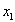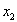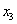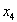02 3 1 0 24 02 1 0 1 16

Table 1: Initial tableau of the example Product Planning

 6 7 0 0 b70 1 1/2 -1/2 4 61 0 -1/4 3/4 60 0 -2 -1 64

Table 2 : Optimal Solution of the example Product Planning

2.3.1 Economic Interpretation of theValues
First, consider the economic interpretation of thevalues, the bottom row of the simplex table. Recall that Zj is the opportunity cost of introducing one unit of variable j into the solution-the cost of replacing or substituting for other solution variables. Since cj is the unit profit, thevalue is the net profit (profit minus opportunity cost) resulting from introducing one unit of j into the solution.

In our example first consider thevalue associated with a slack variable, such as variable x3 in Table 2. Variable x3 is the slack variable associated with the first constraint, the limit on time available for machine 1. In the optimal solution in Table 2,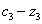has a value of -2, meaning that introducing one unit of x3 (i.e., one hour of slack time) into the solution will decrease profit by \$2. Note that introducing one unit of slack into the solution is effectively the same as reducing the capacity of machine 1 by one hour. Hence, the; values for slack variables associated with constraints can be considered as the marginal values associated with changes in capacity on that constraint. We called these marginal values dual prices. When a decision variable is not included in the optimal solution, thevalue represents the cost of forcing one unit into the solution.

2.3.2 Right-Hand Side Ranges

The interpretation of the dual price of x3 in Table 2 is that one additional hour of machine time on machine 1 would be worth \$2. We might ask the following question: If we could buy additional hours of machine 1 time for less than \$2, could we increase our profit indefinitely by adding more hours?

We can answer this question by examining the x 3 column in Table 2. Recall the meaning of the coefficients in the table; if we were to introduce one unit of x3, it would replace 1/2 unit of x2, and 1/4 unit of x1, (i.e., it would actually add 1/4 unit of x1).

The question posed above, then, can be answered by considering how much x3 can change before a change in the solution mix will occur.

If we enter positive x3; into the solution, the interpretation is that additional slack hours are made available on machine 1-and thus, those hours to be used for production are reduced. In the table, the process of introducing x3 is the same as doing an iteration in the simplex procedure with x3 as the entering variable. We divide the "Solution values" column by the amount in the comparable row of the x3 column (Table 3). The smallest nonnegative number thus obtained gives the number of units of x3 :

 0 Solution values B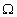71/2 4 8 6-1/4 6 -24-2 64

Table 3: Entering positive x3

Here, the x2 row is the only positive value and hence is also the smallest nonnegative value. This means that eight units of x3 can be introduced before variable x2 goes out of the solution. In terms of the problem, this means that we can cut back on a maximum of eight hours of those available on machine 1 before production of A product stops.

We can also consider adding negative x3. This means that we make additional hours available on machine 1. In order to consider introducing negative x3 into our table, we can proceed as above, except that we must multiply all the values in the x3 column by (- 1) before going to the next step in the simplex procedure. Thus, we would have Table 4:

 0 Solution values B7-1/2 4 -8 61/4 6 24-2 64

Table 4: Entering negative x3

As before, the smallest nonnegative value determines how many units of negative x 3 can be introduced. Here, it is 24; after adding 24 additional hours of time (i.e., negative x3) on machine 1, variable x1, goes to zero and out of the solution.

The two steps can be combined into one. Look at the ratios of the "Solution values" column to the slack variable column. The smallest nonnegative ratio determines the amount of decrease; the smallest (in the sense of being closest to zero) nonpositive ratio determines the amount of the increase. For purposes of this rule, a ratio of zero divided by a positive number is considered positive, and zero divided by a negative number is negative.

We have derived a range of values relative to the amount of time available on machine 1. We started out with 24 hours available. When, we found that we could take away 8 hours before the solution mix changed, or we could add 24 hours before the solution mix changed. Hence, we have a range of 16 to 48 for hours available on machine 1, over which the basic solution mix does not change. Recall that the dual price for a marginal hour of machine 1 time was \$2 (thevalue). This value holds over the range we have just determined. In short, machine time on machine I has a value of \$2 per hour over a range of available hours from 16 to 48 (assuming machine 2 hours remain fixed at 16).

Note that 16 to 48 hours is a range within which the solution mix remains the same. That is, the list of solution variables does not change. The actual solution values will change as more or less time is available on machine 1. Also, total profit will change at a rate of \$2 per hour (the marginal value for x3) for increments of machine 1 time.

We can do the same type of analysis on the time available on machine 2. Variable x4 is the slack variable associated with machine 2. Look at the ratio of the "Solution Values" column to the slack variable column for x4:

 0 Solution values B7-1/2 4 -8 63/4 6 8-2 64

Table 5: Entering x4

As indicated above, the smallest nonnegative value determines the amount of decrease; the smallest (in the sense of being closest to zero) nonpositive ratio determines the increase. Machine 2 has 16 hours available and both the increase and decrease in this case is 8, or a range from 8 to 24 hours.

A summary of these results is shown in Table 6.

 Resource Machine hours Available Dual price Range over which dual price is valid (original RHS) Lower Limit Upper Limit Machine 1 24 2 16 48 Machine 2 16 1 8 24

Table 6: RHS Ranges

In some cases, all of the available capacity of a constraint is not used, and the slack variable will have a positive value in the solution. The dual price in such cases is zero-all of the available capacity is not being used, so additional capacity has no value. In that case, there is no upper limit on the range within which the zero dual price holds. But how much could the capacity be reduced before the solution changed? The answer is simple-if there are, for example, eight hours of slack in a given constraint, then capacity can be reduced by this eight hours before the solution is forced to change .

2.3.3 Changes in the Prices

Another major concern to managers is the sensitivity of the linear programming solution to changes in prices: that is, to changes in the per-unit profits (or costs) of variables in the objective function.

In the case of variables that are not in the solution, determining this sensitivity is relatively easy. Recall that the Zj measures the opportunity cost of introducing one unit of the particular variable into the solution. If the variable is not in the optimum solution, it means that its profitability (cj) is not as great as the opportunity cost Zj . In other words,is negative (This is true if we are maximising. If we are minimising, then the variable will come into the solution if the cost drops below Zj.) To come into the solution, the profit must exceed Zj. This is the upper limit before a change occurs. On the other hand, since the variable is not currently profitable enough to be in the solution, any decrease in per unit profit will not change its status.

In summary, for a variable not in the optimum solution, the price ranges are:

Lower Limit: no

Current Value: cj

Upper Limit: Zj

Within these limits, there is no change in the optimal solution.

For variables that are already in the solution, determining the sensitivity to per-unit profit changes is not so simple. The dual to a linear programming problem is a complementary problem, the details of which will be explained. The same kind of analysis used to determine the right-hand side ranges can be used on the dual problem to determine the profit coefficient ranges.

Consider variable x1, which had an original price of \$6 and is a solution variable. We divide the coefficients of therow in Table 2 by the coefficients of the x1, row This is the dual problem equivalent of dividing the "Solution values" column by the slack variable column used for right-hand side ranging. Then (see Table 7), the smallest nonnegative ratio obtained determines the amount of the increase in price, and the smallest nonpositive (closest to zero) ratio determines the decrease in price (exclude thex1, column from these calculations).

 7 0 060 -1/4 3/40 -2 -1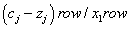/ 8 -4/3

Table 7 : Possible changes in c1 Price

Thus, the possible profit increase is \$8 and the decrease is \$4/3 for variable x1. That is, the per-unit profit can range from \$6 - \$ 1.33 = \$4.67 to \$6 + \$8 = \$14 without any change in the solution.

A similar analysis can be done for variable x2, which price can vary from \$3 to \$9. Recall that the solution of this problem called for the production of six units of x1 and four units of x2. That this solution will not change unless the profit from a unit of x1, drops below \$4.67 per unit, or increases to above \$14 per unit. Similarly, the solution will not change unless the x2 profit per unit falls below \$3 or increases above \$9. Within the ranges specified by the objective function values, both the solution mix and the solution values remain the same. Total profit changes, however, when the unit profits (individual values) change.

The kind of information contained in sensitivity analysis is very valuable to management. The prices are usually subject to change from time to time. If a change is within the range determined by the sensitivity analysis, then there is

no effect upon the optimum solution. If the change is outside the range, a new solution is needed, and the programming problem must be re-solved.

The linear programming problem is set up as a decision problem under certainty. Few problems in the real world are truly certain. Often, many factors are unknown and must be estimated using the best judgement available. The use of sensitivity analysis helps to show over what ranges the solution is and is not subject to change. As such, it is an important adjunct to the interpretation of the solution of a linear programming problem.

2.3.4 Summary
Detail examination of the optimal results including sensitivity analysis is at least as important as the solution itself. The final solution gives many answers useful for managers decision making and represents the real power and value of LP.

Shadow prices can be useful in identifying bottlenecks or constraints that are cost and might be profitably changed. Shadow prices can also be useful in evaluating products.

The ranges, both for the RHS coefficients and for the objective function coefficients, provide important information in interpreting the LP solution. The RHS ranges determine the limits within which the shadow price holds for each constraint. The objective coefficient ranges determine limits within which the solution remains the same.

The sensitivity analysis should be included in software product used for solving LP models. The user must first know it the analysis is really available and that he or she must learn how to use and interpret the computer output.

2.4 The Dual Problem

Every linear programming problem we have solved has been of the type designated as primal, the primal being the first problem to which our attention is generally directed. Each primal problem has a companion problem called the dual. The dual has the same optimum solution as the primal, but it is derived by an alternative procedure. The analysis of this procedure may be instructive for several types of decision problems.

Frequently, the economic problem being solved as the primal involves the maximisation of an objective function (for example, profits), subject to constraints that are frequently of a physical nature (such as hours of machine time). In this type of situation, the dual involves the minimisation of total opportunity costs, subject to the opportunity cost (or equivalently, the value) of the inputs of each product being equal to or greater than the unit profit of the product.

Example : Primal Problem

There are five steps to obtain the dual from the primal problem:

1. If the objective function is maximised in the primal, the objective function of the dual is minimised. If the objective function of the primal is a profit equation, the objective function of the dual will be a cost equation.

2. The coefficients of the variables of the cost equation (the dual objective function) are the right-hand side constants of the primal constraints.

The constants for the dual constraints are obtained from the profit function (the objective function) of the primal.

4. Transposing the coefficients used in the primal forms the dual constraints. The first column of A becomes the first row of AT, and the second column of A becomes the second row of AT.

5. If we are maximising a primal objective function and if the constraints of the primal are "less than or equal to," the constraints of the dual will be "greater than or equal to."

If this entire procedure is performed on the dual, the original (primal) problem will be obtained. Thus, the dual of the dual is the primal.

These rules require the primal problem to be either a maximisation problem with all "less than or equal to" constraints, or a minimisation problem with all "greater than or equal to" constraints. If an LP problem does not have one of these forms, multiplying the objective function or the constraints by (- 1) and writing equality constraints as two inequality constraints will enable it to be placed in one of the forms required by the rules listed above.

Example: Dual Problem

The economical interpretation of the dual solution is of considerable importance and usefulness.

An important fact is that the dual of dual is the original model. The values of the dual variables of the dual problem are the optimal values of the original models variables. I f the original model has m constraints and n variables, its dual has m variables and a constraints. If the number of constraints is much greater than the number of Variables, solving the dual problem is easier. Unless the computational saving are significant, you should solve the model that interests you, not its dual. Sensitivity analysis and model modification are easier on the original model.

2.4.1 Summary

Although the primal and dual problems use the same numerical values, the relationship between the two is not obvious. If one model is ill-behaved, then so is the other. If one model has a finite optimal solution, then so does the others and the optimal objective function values are equal. The are relationship between constraints in one model and variable in the other. The interpretation of the dual constraints should help clarify the relationship between the primal and dual problems. Solving the dual problem brings answers to another questions than the primal solution. The dual theory is a base for sensitivity analysis of the prices.